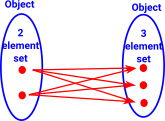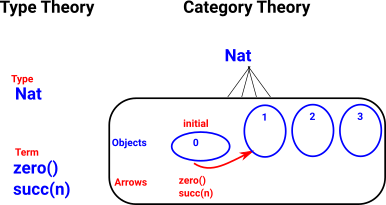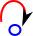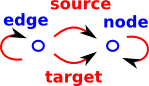# Maths - Concrete Categories

On these pages we look at concrete categories. Concrete categories are a set together with some form of 'structure' such as functions and operations within the category. (ref nLab).

Although category theory requires different assumptions and ways of thinking than other approaches such as set theory and type theory I believe we need to be able to translate between the two. It seems to me that, to really understand a mathematical topic, it helps to be able to approach it from many directions.

Also it may not be practical to do everything in category theory. For instance, if an engineer is calculating the loading of a bridge, they probably don't want to calculate it 'up to isomorphism'.

So lets look at how objects and arrows in category theory may, or may not, relate to types and terms in type theory.

In type theory we have types and terms (and perhaps a higher level type constructor) so a sort of three layer hierarchy. In category theory:

• The type constructor might correspond to the category (FinSet category)
• A type might correspond to an object in category theory.
• A term (element of the set) is not explicitly referenced in category theory (we only look at the external properties, not what's inside it).

Consider finite sets:

### FinSet

 In a finite set category objects are: The empty set. A one element set A two element set A three element set ...The arrows are the mappings between these objects.

 Of course category theory does not look inside objects or arrows to see the elements but, to try to give better intuition, lets make an exception in this diagram:.For further discussion of the properties of sets in category theory see this page.

### Natural Numbers

Not all catergories relate to type theory in the same way as FinSet.

 In the case of natural numbers: Types map to objects. Terms map to arrows.### Monoid

In set there were few restrictions on arrows (apart from no arrows to empty set).

However when we add extra structure to the set this puts constraints on what arrows we can have.

We can think of a monoid as a set with some extra structure . So we can start with an underlying set then represent the monoid operation by arrows which constrain what is allowed to comply with the axioms.

 However the monoid category is more usually modeled as a single object with multiple arrows from and back to itself (endo-functors).### Finite Categories

Name Diagram Description
0   No objects
No arrows
1One object
The only arrow is the arrow back to itself (identity arrow).

For instance: if the object is 'set' then the whole thing is a monoid.

2Two objects

here are some examples:1+1 that is the disjoint union of two single objectsThis could be an index categoryThis could be a graph
3Three objects.
The arrow from first to last is implies by the composition law.

Here we avoid loops (exept identity) which would mean that something else is going on (such as isomorphism or adjunctions).

### Categories with binary operation and identity element

 single objects multiple objects -> composition law no composition law invertible Groups Groupoids all morphisms are isomorphisms Graph non-invertible Monoids Categories Directed Graph

## Illustrating Category Constructions

In order to get an intuitive understanding of category theoretic constructions I find it helps to use examples from the simplest concrete categories to show what is going on internally, especially if it can be illustrated graphically. Of course, we are not really supposed to look inside categories, but I think its necessary to get an intuitive understanding.

Often sets are the simplest category to show whats going on.

If sets don't have enough structure the a pre-order (see this page) is often a good example to use (on this page I have tried to use preorders to show the idea of adjunctions). pre-orders are good because of the graphical nature of Hasse diagrams.

Pre-orders are also good because they are a specific (less general) case of categories in that, like categories, they are reflexive and transitive:

#### reflexivea≤a

If a relationship is reflexive then there is always an arrow from an element of a set to itself. Although when drawing the Hasse diagram we may not draw it to avoid clutter.

#### transitiveif a≤b and b≤c then a≤c

This is like composing functions. That is: if the is an arrow from 'a' to 'b' and an arrow from 'b' to 'c', then we can attach the tip of the first arrow to the second arrow to get an arrow from 'a' to 'c'.

xOn these pages we look at concrete categorories. A definition of concrete categorories is a set together with some form of 'structure' such as functions and operations within the category. (ref nLab)

Where I can, I have put links to Amazon for books that are relevant to the subject, click on the appropriate country flag to get more details of the book or to buy it from them.The Princeton Companion to Mathematics - This is a big book that attempts to give a wide overview of the whole of mathematics, inevitably there are many things missing, but it gives a good insight into the history, concepts, branches, theorems and wider perspective of mathematics. It is well written and, if you are interested in maths, this is the type of book where you can open a page at random and find something interesting to read. To some extent it can be used as a reference book, although it doesn't have tables of formula for trig functions and so on, but where it is most useful is when you want to read about various topics to find out which topics are interesting and relevant to you.